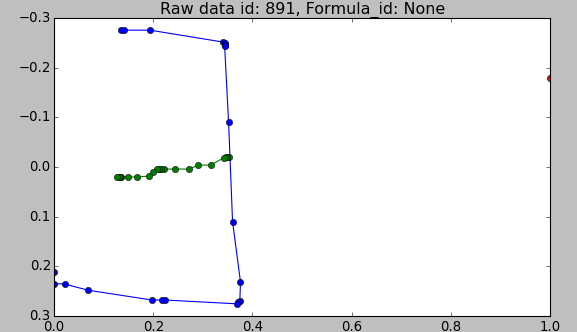# Preprocessing¶

Preprocessing algorithms.

Each algorithm works on the HandwrittenData class. They have to be applied like this:

>>> a = HandwrittenData(...)
>>> preprocessing_queue = [ScaleAndShift(),
StrokeConnect(),
DouglasPeucker(epsilon=0.2),
SpaceEvenly(number=100)]
>>> a.preprocessing(preprocessing_queue)

class hwrt.preprocessing.DotReduction(threshold=5)

Reduce strokes where the maximum distance between points is below a threshold to a single dot.

class hwrt.preprocessing.DouglasPeucker(epsilon=0.2)

Apply the Douglas-Peucker stroke simplification algorithm separately to each stroke of the recording. The algorithm has a threshold parameter epsilon that indicates how much the stroke is simplified. The smaller the parameter, the closer will the resulting strokes be to the original.

class hwrt.preprocessing.RemoveDots

Remove all strokes that have only a single point (a dot) from the recording, except if the whole recording consists of dots only.

class hwrt.preprocessing.RemoveDuplicateTime

If a recording has two points with the same timestamp, than the second point will be discarded. This is useful for a couple of algorithms that don’t expect two points at the same time.

Scale a recording so that it fits into a unit square. This keeps the aspect ratio. Then the recording is shifted. The default way is to shift it so that the recording is in [0, 1] × [0,1]. However, it can also be used to be centered within [-1, 1] × [-1, 1] around the origin (0, 0) by setting center=True and center_other=True.

class hwrt.preprocessing.SpaceEvenly(number=100, kind='cubic')

Space the points evenly in time over the complete recording. The parameter ‘number’ defines how many.

class hwrt.preprocessing.SpaceEvenlyPerStroke(number=100, kind='cubic')

Space the points evenly for every single stroke separately. The parameter number defines how many points are used per stroke and the parameter kind defines which kind of interpolation is used. Possible values include cubic, quadratic, linear, nearest. This part of the implementation relies on scipy.interpolate.interp1d.

class hwrt.preprocessing.StrokeConnect(minimum_distance=0.05)

StrokeConnect: Detect if strokes were probably accidentally disconnected. If that is the case, connect them. This is detected by the threshold parameter minimum_distance. If the distance between the end point of a stroke and the first point of the next stroke is below the minimum distance, the strokes will be connected.

class hwrt.preprocessing.WeightedAverageSmoothing(theta=None)

Smooth every stroke by a weighted average. This algorithm takes a list theta of 3 numbers that are the weights used for smoothing.

class hwrt.preprocessing.WildPointFilter(threshold=3.0)

Find wild points and remove them. The threshold means speed in pixels / ms.

hwrt.preprocessing.euclidean_distance(p1, p2)

Calculate the euclidean distance of two 2D points.

>>> euclidean_distance({'x': 0, 'y': 0}, {'x': 0, 'y': 3})
3.0
>>> euclidean_distance({'x': 0, 'y': 0}, {'x': 0, 'y': -3})
3.0
>>> euclidean_distance({'x': 0, 'y': 0}, {'x': 3, 'y': 4})
5.0

hwrt.preprocessing.get_preprocessing_queue(preprocessing_list)

Get preprocessing queue from a list of dictionaries

>>> l = [{'RemoveDuplicateTime': None},
{'ScaleAndShift': [{'center': True}]}
]
>>> get_preprocessing_queue(l)
[RemoveDuplicateTime, ScaleAndShift
- center: True
- max_width: 1
- max_height: 1
]

hwrt.preprocessing.print_preprocessing_list(preprocessing_queue)

Print the preproc_list in a human-readable form.

Parameters: preprocessing_queue : list of preprocessing objects Algorithms that get applied for preprocessing.Effect of a wild point on scale and shift

Handwritten Data

#### Next topic

Data Multiplication